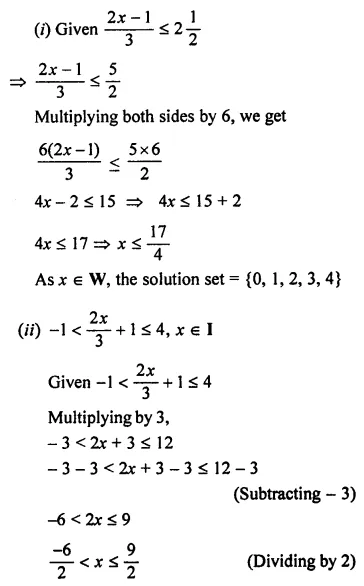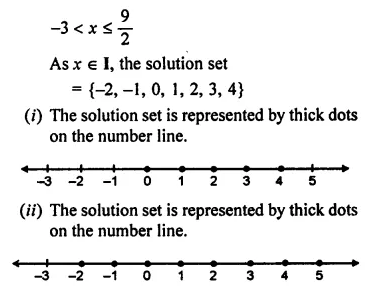# ML Aggarwal Class 7 Solutions for ICSE Maths Chapter 9 Linear Equations and Inequalities Check Your Progress

## ML Aggarwal Class 7 Solutions for ICSE Maths Chapter 9 Linear Equations and Inequalities Check Your Progress

Question 1.
Solve the following equations :
(i) 2 (x – 5) + 3 (x – 2) = 8 + 7 (x – 4)Solution: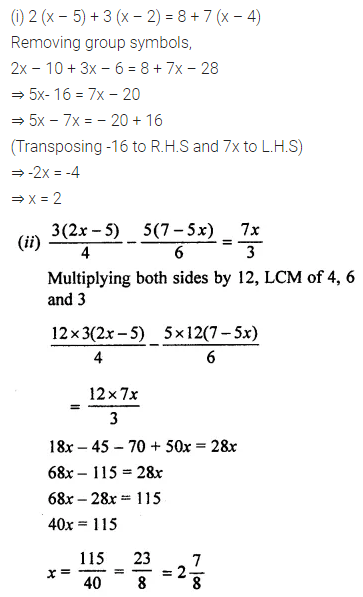Question 2.
A number exceeds its three-fifth by 22. Find the number.
Solution: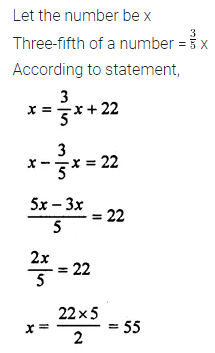Question 3.
When 9 is added to twice a number, the result is 3 more than thrice the number. Find the number.
Solution:Question 4.
The ten’s digit of a two digit number is twice the unit’s digit. The sum of the number and its unit’s digit is 66. Find the number.
Solution: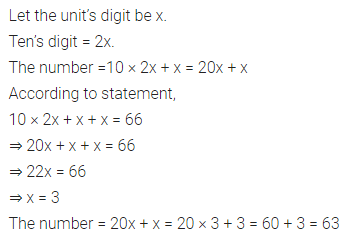Question 5.
A student bought some pens at ₹ 8 each and some pencils at ₹ 1.50 each. If the total number of pens and pencils purchased is 16 and their total cost is ₹ 50, how many pens did he buy?
Solution: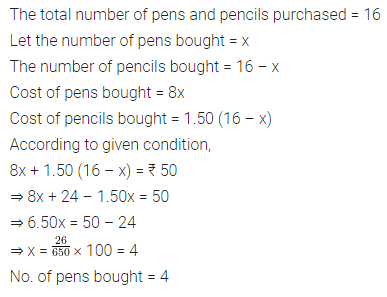Question 6.
Arvind is eight years older than his sister. In three years, he will be twice as old as his sister. How old are they now?
Solution:Question 7.
The angles of a triangle are in the ratio 1 : 2 : 3. Find their measure in degrees.
Solution:Question 8.
Solve the following inequations and represent their solution on a number line:Solution: